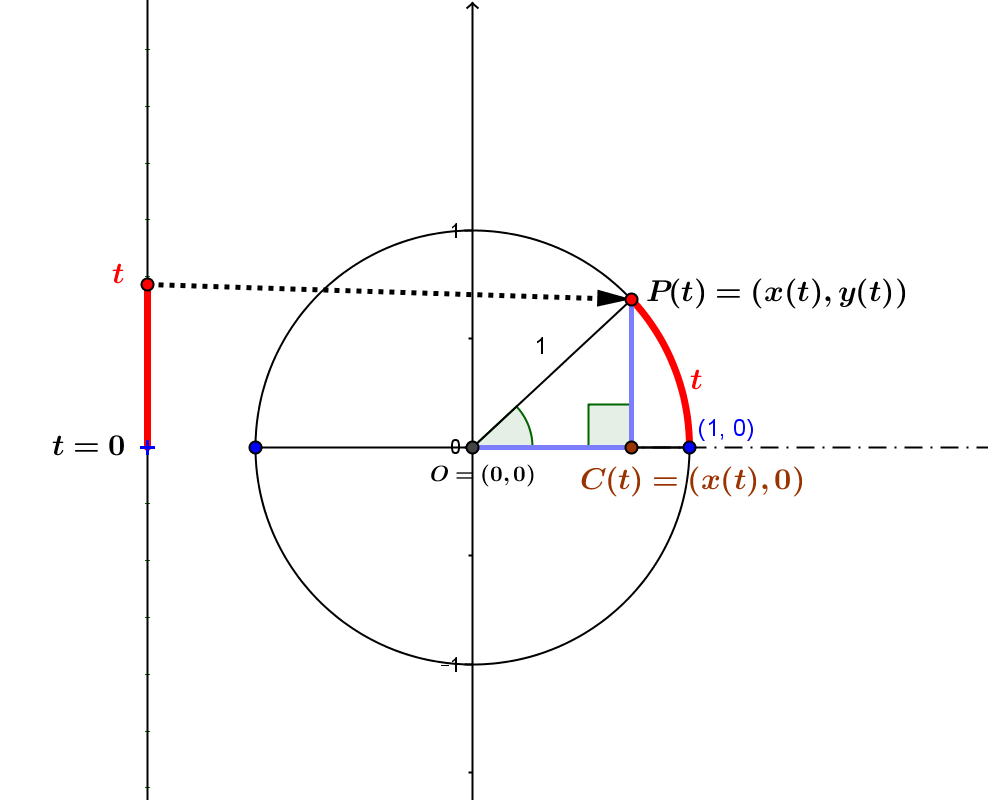The trigonometric functions are defined in two related approaches:
(i) Right Triangle Trigonometry.
(ii) Unit Circle Trigonometry.

(i) In right triangle trigonometry, six functions are defined with acute angles, right triangles, and the theory of similar of triangles: sine, cosine, tangent, cotangent, secant and cosecant.
In most courses on triangle trigonometry, the main attention is paid to the sine, cosine, and tangent functions. These three functions are the most frequently used for the study of triangles- especially with problems of solving triangles using the laws of sines and cosines and the use of tangents to measure inclination of an angle.
In the study of trigonometry applied to general triangles, treating obtuse angles becomes relevant. The definitions of the trigonometric functions are extended to angles of degree measures between 0 and 180. Making sense of the laws of sines and cosines and solving triangles are central applications for this extension.
For our treatment of triangle trigonometry we consider all six functions as "core".
Note: The theory of similar right triangle shows that the value of any one of these functions will determine the angle and thus the other five functions, we could consider the sine function as the core of all trigonometric functions for right triangles.
Details: Right Triangle Trigonometric Functions(ii) The unit circle approach to the trigonometric functions comes from considering central angles in the geometry of circles- in particular looking at a unit circle centered at the point \$O=(0,0)\$ in cartesian coordinate plane.
Central angles with  the positive horizontal axis as the initial ray are determined by an arc length, \$t\$- the radian measure of the angle. This arc also determines a right triangle by the center \$O\$, the endpoint of the arc, \$P(t)=(x(t), y(t)\$, and the point on the horizontal axis, \$C(t)=(x(t),0)\$. The right angle of the triangle is at the point \$C(t)\$.
Since the triangle \$OC(t)P(t)\$ has a unit length for the measure of its hypotenuse, the right triangle trigonometric functions of cosine and sine correspond to the coordinates of \$P(t)\$. This correspondence is used to extend the definition of these trigonometric functions to all real numbers. The use of the coordinates related to the unit circle also gives a sensible way to visualize the values of all the trigonometric functions as lengths of line segments, with the easiest line segment visualizations being connected to the sine, cosine, and tangent functions.
Details: Unit Circle Trigonometric Functions